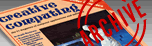`CREATIVE COMPUTING VOL. 9, NO. 1 / JANUARY 1983 / PAGE 250`

 Colorful circles. (computer graphics in color) Alan Foxx. Colorful Circles Circle Fill is a program designed to allow the Apple II to fill in circles. See Listing 1. The program first draws a circle, saving each point into two arrays. The x-coordinate of each point is saved in the array AX. They y-coordinate of each point is saved in thearray AY. For example, the x-coordinate of the first point is saved in AX(1). They y-coordinate of the first point is saved in AY(1). The x-coordinate of the second point is saved in AX(2). They y-coordinate of the second point is saved in AY(2) and so on. The outer perimeter of the circle is composed of 100 dots (see Figure 1), therefore AX(100) and AY(100) contain the value of the coordinates of the 100th and last dot. After drawing the circle, the program fills the circle in. It accomplishes this by connecting each dot on the outer perimeter of the circle to all the others. Lines are drawn connecting the first dot to the second dot, the first dot to the third dot, the first dot to the fourth dot and so on. This routine occurs in lines 230 thru 260. To speed up this process you may change line 230 to read: 230 FOR J = 1 TO 100 STEP 10 You may want to make some changes in the program. For example, to change the color of the circle, change line 120. To change the diameter of the circle change the value of DIAM in line 130. To move the circle to another position on the screen, change the variables XP and YP in line 130. One word of caution: the entire circle must be on the screen. Therefore do not make the circle too big or you will get an illegal quantity error in line 200. To make an ellipse change line 180 to read: 180 AX(C) = R * SIN (J) / 3 + XP or change line 190 to read: 190 AY(C) = R * COS (J) / 3 + YP The Colorful Circle The Colorful Circle program in Listing 2 produces some very interesting patterns using the circle formula, and the random number generator. Like Circle Fill, this program draws a circle with 100 dots and saves each dot into two arrays. Then, changing colors each time, lines are drawn connecting each dot to every other dot to produce interesting patterns. Figure 3 shows some examples of what this program can do. Four Colorful Circles The Four Colorful Circles program in Listing 3 is very similar to the Colorful Circle program. It incorporates the following changes: Instead of one circle there are now four on the screen, and the number of dots is decreased from 100 to 50. Another change was that thecolors are only 1 and 2 (green and blue) instead of random. The program uses a symmetry algorithm. In other words, when the computer plots a dot at (x, y) it will also plot three more dots at (279-x, y), (x, 159-y), and (279-x, 159-y). Figure 4 shows some examples of what the Four Colorful Circles program can do. Table: Listing 1. Table: Listing 2. Table: Listing 3. Photo: Figure 1. Circle before fill-in. Photo: Figure 2. Circle after fill-in. Photo: Figure 3. Examples of what the Colorful Circle program can do. Photo: Figure 4. Examples of what the Four Colorful Circles program can do.